American Journal of Physics and Applications
Volume 5, Issue 1, January 2017, Pages: 1-5

The Effect of Temperature on Conductivity of Conductors and Superconductors

Amel Abdallah Ahmed Elfaki1, *, Abdelnabi Ali Elamin2, Mubarak Dirar Abd-Alla1,
Rawia Abd Elgani1, Ali Suliman Mohammed1, Alaa Ahmed Mohammed1, Sawsan Ahmed Elhouri3

1Department of Physics, College of Science,Sudan University of Science and Technology, Khartoum, Sudan

2Department of Physics, College of Science and Technology,Omdurman Islamic University, Omdurman, Sudan

3Department of Physics, College of Applied and Industrial Science,University of Bahri, Khartoum, Sudan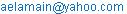(A. A. Elamin)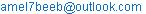(A. A. A. Elfaki)

*Corresponding author

Amel Abdallah Ahmed Elfaki, Mubarak Dirar Abd-Alla, Rawia Abd Elgani, Ali Suliman Mohammed, Alaa Ahmed Mohammed, Abdelnabi Ali Elamin, Sawsan Ahmed Elhouri. The Effect of Temperature on Conductivity of Conductors and Superconductors. American Journal of Physics and Applications. Vol. 5, No. 1, 2017, pp. 1-5. doi: 10.11648/j.ajpa.20170501.11

Received: November 26, 2016; Accepted: January 6, 2017; Published: February 13, 2017

Abstract: The thermal conductivity coefficient has been studied for three types of wires. Pure copper wire, 15cm long copper coated and 50cm long copper coated. In order to compare thermal conductivity for these wires and to recognize the meaning of superconductivity and the effect of temperature on conductivity of conductors and superconductors. The thermal conductivity coefficient has been calculated and the resistivity also has been conducted. The obtained result agrees with theoretical consideration.

Keywords: Superconductivity, Thermal Conductivity Coefficient, Copper

Contents

1. Introduction

Every solid has its own characteristic energy band structure. In order for a material to be conductive, both free electrons and empty states must be available. Metals have free electrons and partially filled valence bands, therefore they are highly conductive. Semimetals have their highest band filled. This filled band, however, overlaps with the next higher band, therefore they are conductive but with slightly higher resistivity than normal metals Examples: arsenic, bismuth, and antimony.

Insulators have filled valence bands and empty conduction bands, separated by a large band gap Eg (typically>4eV), they have high resistivity. Semiconductors have similar band structure as insulators but with a much smaller band gap. Some electrons can jump to the empty conduction band by thermal or optical excitation. Eg =1.1 eV for Si, 0.67 eV for Ge and 1.43 eV for GaAs [1,2].

Superconductivity in (SC) physics is the phenomenon that occurs in certain materials when cooled to very low temperatures close to absolute zero (Zero Kelvin), where superconductors allow the passage of electricity through without almost any electrical resistance [3, 4].

Superconductivity was discovered in 1911 in the Leiden laboratory of Kimberling Ones when a so-called "blue boy" (local high school student recruited for the tedious job of monitoring experiments) noticed that the resistivity of Hg metal vanished abruptly at about 4K . Although phenomenological models with predictive power were developed in the 30’s and 40’s, the microscopic mechanism underlying superconductivity was not discovered until 1957 by Bardeen Cooper and Schrieffer. Superconductors have been studied intensively for their fundamental interest and for the promise of technological applications which would be possible if a material which super conducts at room temperature were discovered. Until 1986, critical temperatures (’s) at which resistance disappears were always less than about 23K. In 1986, Bednorz and Mueller published a paper, subsequently recognized with the 1987 Nobel prize, for the discovery of a new class of materials which currently include members with’s of about 135K .

The SC disappearance below a certain critical temperature was tackled by many researchers. Here one reed to modify the temperature resistance relation for ordinary medic to find the critical temperature beyond with resistance vanishes.

According to Ohm’s law the voltage (V) set up across a solution is proportional to the flowing current (I):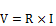(1)

R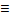Resistance (ohm, Ω), VVoltage (volt, V), ICurrent (ampere, A).

The resistance (R) is a constant of proportionality and can be calculated with the measured current flow if a known voltage is applied: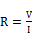(2)

Conductance (G) is defined as the inverse of resistance: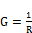(3)

Gconductance (Siemens, S) .

The resistivity ρ is defined by scattering events due to the Imperfections and thermal vibrations. Total resistivity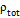can be described by the Matthiessen rule: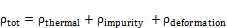(4)

Where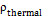: is a thermal vibrant,is an impurity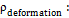is deformation-induced defects .

There are three contributions top: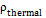due to phonons (thermal),due to impurities,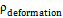due to deformation .

Resistivity rises linearly with temperature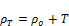(5)

2. Temperature Dependent Superconductor Resistance

The main common physical parameter that relates conductor to Sc is the temperature. In ordinary conductor temperature increase resistance according to the relation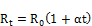(6)

2.1. Critical Temperature

The phase transition from normal into the superconducting state is a second order transition, occurring at a temperature called the critical temperature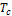.

2.2. Order Parameter

In general, pairing is possible for some pair the mechanism if single particle energies corresponding to the states k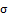and k`σ` are degenerate, since in this case the pairing interaction is most attractive. In the BCS case, a guarantee of this degeneracy for k ↑ and −k ↓ in zero fields is provided by Kramer’s theorem, which says these states must be degenerate because they are connected by time reversal symmetry. They are connected by time reversal symmetry. However, there are other symmetries: in a system with inversion symmetry, parity will provide another type of degeneracy, so k ↑, k ↓, −k ↑ and −k ↓ are all degenerate and may be paired with one another if allowed by the pair interaction .

2.3. Penetration Depth

Is a very thin layer through which high-current (supercurrent) on the surface of a material with high conductivity and by which high-conductivity material may cancel the magnetic field inside through the consolidation of this constant current[1,2].

2.4. Coherence Length and the Cooper-Pair Size

In many of the scientific literature they consider that the distance between the two electrons in a Cooper pair (size of the Cooper pair) is exactly the same so-called correlation length. But there is a difference between the two concepts. As the size of a pair Cooper be linked aftershock waveform pair Cooper (the waveform is a physicist, a term found in quantum mechanics, where a particle is described by that of the waveform, and gives the following relationship (-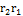ψ where the difference between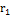and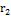represent relative coordinates, as the case of high conductivity is a quantum state occur on the macroscopic level.) and is independent of temperature .

The length of the correlation parameter defines the change in rank (order parameter) of condensate super-conductivity. Where the parameter defines Rank: that the waveform of the output of the intensification of high-conductivity, in other words, you can magnetic fields influence into the ultra-carrier and the form of filaments microscopic filaments called vortices vortices) (at regular three-network and each of these vortices is made up of ordinary nuclei and form that inside the magnetic field is large and surrounded by high conductivity zone, and an approximation of a long cylinder with a parallel axis of the magnetic field outside as inside the roller be parameter rank non-existent, and thus the radius of the drum represents the so-called rank along bonding. and the length of interdependence be continued on the temperature .

3. Methodology and Practical

The resistance-change factor per degree Celsius of temperature change is called the temperature coefficient of resistance. This factor is represented by the Greek lower-case letter "alpha" (α). The resistance of all substances varies with temperature. This temperature resistance dependence has a bearing on electronic circuits in many ways. In most cases the resistance increases with temperature, but in some it falls. As a result it is often necessary to have an understanding of the resistance temperature dependence.

3.1. Equipment and Materials

Standard Resistance of (S =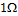), Galvanometer (G), DC power supply, 2 holders, thermometer, calorimeter, heater, Welding wire, Wheatstone bridge (1m), copper coil, micrometer.

3.2. Theory(7)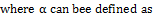(8)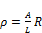(9)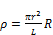(10)

Where: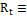The metal resistance at a temperature t/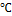,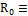The metal resistance at a temperature 0/.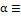Thermal coefficient,Temperature,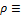Resistivity.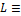Length of the wire.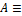Cross Sectional area,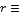The radius of the wire.

3.3. The Procedure

The circuit was connected as shown in figure 1 then heater has been switched on to 100 degree Celsius the unknown resistance, using wheat stone bridge the current was set at zero balance. Then as the temperature increases the (G) starts to lead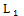represents the length between the wire at wheatstone bridge and the Known resistance (1W) and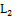represents the length between the wheatstone bridge wire and the unknown resistant of the these wires. Calculated for different temperature in cooling manner the result has been tabulated as in table 1. the previous steps were repeated by taking 15cm of coated Cu wire by welding as in table (2) and then 50cm has been coated by the same way in table 3. the resistivity has been calculated for all samples. The relation between obtained resistances R (T) and temperature T is plotted as in graphs 1, 2 and 3.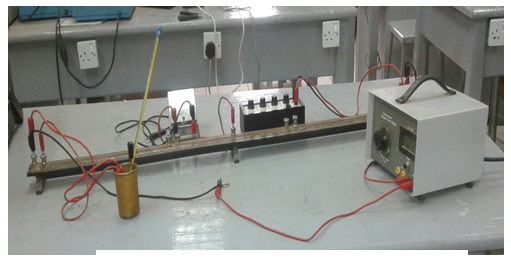Figure 1. The circuit diagram.

4. Results

Table 1. Shows the relationship between the net resistances of copper with the temperature.

 T/°C L1/cm L2/cm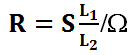90 10.1 89.7 0.112 80 9.2 90.8 0.101 70 9.0 91.0 0.099 60 9.3 90.7 0.103 50 9.0 91.0 0.099 40 8.5 91.5 0.093 30 8.7 91.3 0.095

Table 2. Shows the relationship between the resistances of the coated wire length of 15 cm with temperature.

 T/°C Length (L1/cm Length (L2/cm90 10.3 89.7 0.115 80 9.9 90.1 0.109 70 9.5 90.5 0.105 60 9.1 90.9 0.100 50 8.9 91.1 0.098 40 8.6 91.4 0.094 30 8.0 92.0 0.087

Table 3. Shows the relationship between the resistances of the coated wire length of 50 cm with temperature.

 T/°C L1/cm L2/cm90 8.8 91.2 0.096 80 7.6 92.4 0.082 70 7.5 92.5 0.081 60 7.4 92.6 0.080 50 7.1 92.9 0.076 40 7.1 92.9 0.076 30 7.3 92.7 0.079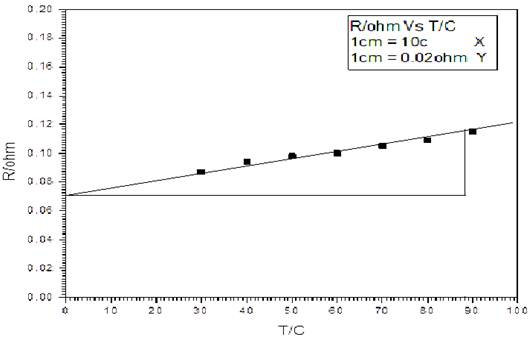Figure 2. Showing the relationship between the net resistances of copper with the temperature.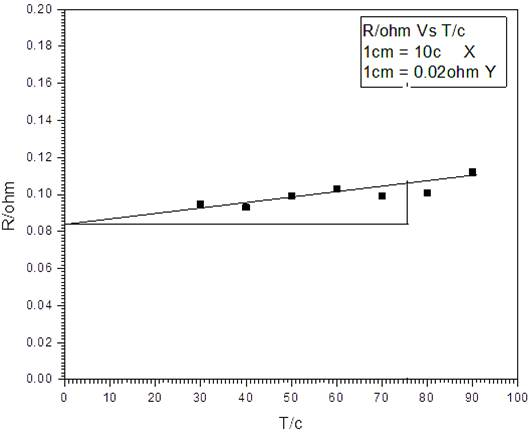Figure 3. Shows the relationship between the resistances of the coated wire length of 15 cm with temperature.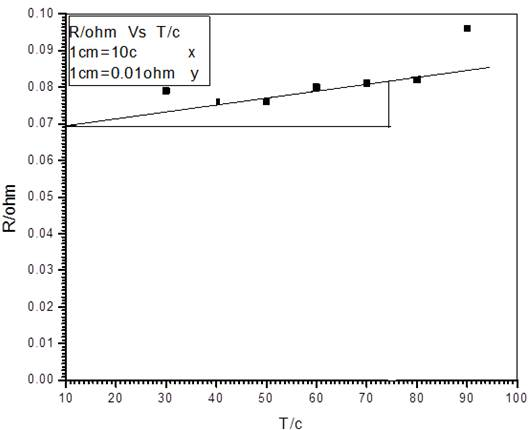Figure 4. Shows the relationship between the resistance of the coated wire length of 50 cm with temperature.

5. Calculations

a)  From equation (8) and figure (2) can obtain: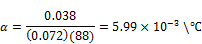And from equation (10)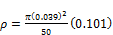=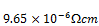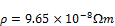b)  From equation (8) and figure (3) can obtain: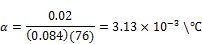And from equation (10)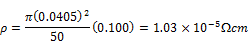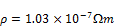c)  From equation (8) and figure (4) one can obtain: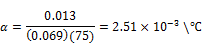And from equation (10)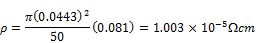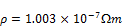Superconductivity and Critical Temperature:

The resistance of Sc Rs vanishes beyond the critical temperature. A cording to equation (1)(11)

One can split this equation to positive superconductivity part Rt and negative part R-, where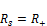(12)

Thus according to equation (11):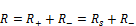(13)

The condition under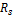vanishes requires R to be negative. Thus according to equations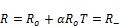(14)

This requires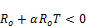(15)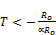(16)(17)

Thus the critical temperature equals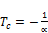(18)

For superconductor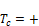(19)

This requires(20)

Also empirically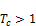(21)

Thus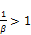(22)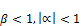(23)

Hence for sc materials the temperature coefficient should be negative and less than untiy.

However for ordinary conductor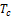does not exist. This requires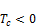(24)

According to equation (18)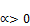(25)

6. Discussion

We note that from the results which obtained in table 1, 2 and 3 there is a relation between temperature and resistance when temperature increases The resistance increases too as in figures 2, 3 and 4.

The intercept of the curves with y-axis gives the value of resistance at room temperature for each type copper wire, 15cm coated with Block Soldering wire and 50cm coated with Block Soldering wire respectively. The room temperature resistance is explained in table 4.

Table 4. The room temperature resistance

 figures 0.072 Figure 2. 0.084 Figure 3. 0.069 Figure 4.

From the table it is clear that the least resistance of the 50cm coated wire while the largest value for 15cm coated.

The thermal coefficient for different wires it is slightly varied due to the weld operation has not effect in thermal conductivity of the wire. And the resistivity of wire calculated was a slight difference, due to variation in the diameter of wire. So that conductivity decreases with increasing resistivity difference.

In previous study it shows that the thermal conductivity coefficientof the graph is calculated to be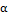= 0.0039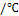while in our study it was calculated to be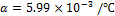due to purity.

7. Conclusions

From the results obtained we note that the thermal conductivity is very sensitive for temperature and the resistivity of Tree copper of wires increased with increasing of temperature. The thermal conductivity coefficientin this study it was calculated to bedue to purity which was compared with previous study is calculated to be= 0.0039.

References

1. Donald R. Askeland, Pradeep P. Fulay, Wendelin J. Wright, 2011, The Science and Engineering of Materials, Sixth Edition, English Book; Illustrated, 921 pages, Cengage Learning.
2. Brian S. Mitchell, January 2004, An Introduction to Materials Engineering and Science for Chemical and Materials Engineers, ISBN: 978-0-471-43623-2, 976 pages.
3. Andrei Mourachkine, 2002, ROOM-TEMPERATURE SUPERCONDUCTIVITY, University of Cambridge, Cambridge, United Kingdom, CAMBRIDGE INTERNATIONAL SCIENCE PUBLISHING.
4. Barakat, M. Me.; Abou-Aly, A. I.; Awad, R.; et al, DEC 15 2015, Mechanical properties of Y3-xNdxBa5-xCaxCu8O18-delta samples, JOURNAL OF ALLOYS AND COMPOUNDS Volume: 652 Pages: 158-166.
5. Simon R. and A. Smith, 1988, Superconductors: Conquering Technology'sNewFrontier (Plenum, New York,. 2 W. A. Little, Phys. Rev. 134, A1416.
6. Joe Khachan and Stephen Bosi,14 Feb 2014, Superconductivity, http://tinyurl.com/k2735qd. 1: 53 PM -. 0 retweets 0 likes. Reply Retweet. Retweeted. Like.
7. United States Hach Company Brand, Copyright 2015 Hach, Radiometer Analytical, P.O. Box 389. Loveland, Colorado 80539-0389. www.Hach.com. Hach Technical.
8. University of Virginia, Dept. of Materials Science and Engineering Nanoscale Materials, 2009, Introduction to Materials Science, Chapter 19 Electrical Properties.
9. Mohammed AA,2016, temperature copper-oxide-based superconductors are solved and used to calculate several Band theory of solids: metals, semiconductors and insulators: superconducting state to suggest that changes in electron Gibbs free energy,
repository.sustech.edu/bitstream/handle/123456789/14335/Research.pdf.
10. Cyrot, M and D. Pavuna, 1992, Introduction to Superconductivity and High-Tc Materials, Singapore: World Scientific, ISBN: 9810201443. Leggett, A. and D. M.
11. nKarl-Heinz Bennemann,John B. Ketterson - 2004 -Technology & Engineering V. Nazarov, Superlattices and Microstructures 25, 1221 (1999). 20 J.B. Ketterson and S.N. Song, Superconductivity, Cambridge University Press, Cambridge.
12. Anderson P. W., 2010, Theory of Superconductivity in Cuprates, Princeton University Press, Princeton, NJ.
13. Robert Schrieffer J., 2007,Technology & Engineering (AIP, New York, 1972), p. 17. 88. P. W. Anderson, the Theory of Superconductivity in the High-Tc Cuprates (Princeton University Press, Princeton, NJ, 1997). 89.

 Contents 1. 2. 2.1. 2.2. 2.3. 2.4. 3. 3.1. 3.2. 3.3. 4. 5. 6. 7.
Article ToolsAbstractPDF(629K)